# graph_tool.draw - Graph drawing and layout¶

## Summary¶

### Layout algorithms¶

 sfdp_layout Obtain the SFDP spring-block layout of the graph. fruchterman_reingold_layout Calculate the Fruchterman-Reingold spring-block layout of the graph. arf_layout Calculate the ARF spring-block layout of the graph. radial_tree_layout Computes a radial layout of the graph according to the minimum spanning tree centered at the root vertex. planar_layout Performs a canonical layout of a planar graph. random_layout Performs a random layout of the graph.

### Graph drawing¶

 graph_draw Draw a graph to screen or to a file using cairo. draw_hierarchy Draw a nested block model state in a circular hierarchy layout with edge bundling. graphviz_draw Draw a graph using graphviz. prop_to_size Convert property map values to be more useful as a vertex size, or edge width. get_hierarchy_control_points Return the Bézier spline control points for the edges in g, given the hierarchical structure encoded in graph t.

#### Low-level graph drawing¶

 cairo_draw Draw a graph to a cairo context. interactive_window Display an interactive GTK+ window containing the given graph. GraphWidget Interactive GTK+ widget displaying a given graph. GraphWindow Interactive GTK+ window containing a GraphWidget.

## Contents¶

graph_tool.draw.random_layout(g, shape=None, pos=None, dim=2)[source]

Performs a random layout of the graph.

Parameters
gGraph

Graph to be used.

shapetuple or list (optional, default: None)

Rectangular shape of the bounding area. The size of this parameter must match dim, and each element can be either a pair specifying a range, or a single value specifying a range starting from zero. If None is passed, a square of linear size $$\sqrt{N}$$ is used.

posVertexPropertyMap (optional, default: None)

Vector vertex property maps where the coordinates should be stored.

dimint (optional, default: 2)

Number of coordinates per vertex.

Returns
posVertexPropertyMap

A vector-valued vertex property map with the coordinates of the vertices.

Notes

This algorithm has complexity $$O(V)$$.

Examples

>>> g = gt.random_graph(100, lambda: (3, 3))
>>> shape = [[50, 100], [1, 2], 4]
>>> pos = gt.random_layout(g, shape=shape, dim=3)
>>> pos[g.vertex(0)].a
array([68.72700594,  1.03142919,  2.56812658])

graph_tool.draw.planar_layout(g, pos=None)[source]

Performs a canonical layout of a planar graph.

Parameters
gGraph

Planar graph to be used.

posVertexPropertyMap (optional, default: None)

Vector vertex property maps where the coordinates should be stored.

Returns
posVertexPropertyMap

A vector-valued vertex property map with the coordinates of the vertices.

Notes

This algorithm has complexity $$O(V + E)$$.

References

straight-line-boost

http://www.boost.org/doc/libs/release/libs/graph/doc/straight_line_drawing.html

chrobak-linear-1995

M. Chrobak, T. Payne, “A Linear-time Algorithm for Drawing a Planar Graph on the Grid”, Information Processing Letters 54: 241-246, (1995), DOI: 10.1016/0020-0190(95)00020-D [sci-hub, @tor]

Examples

>>> g = gt.lattice([10, 10])
>>> pos = gt.planar_layout(g)
>>> gt.graph_draw(g, pos=pos, output="lattice-planar.pdf")
<...>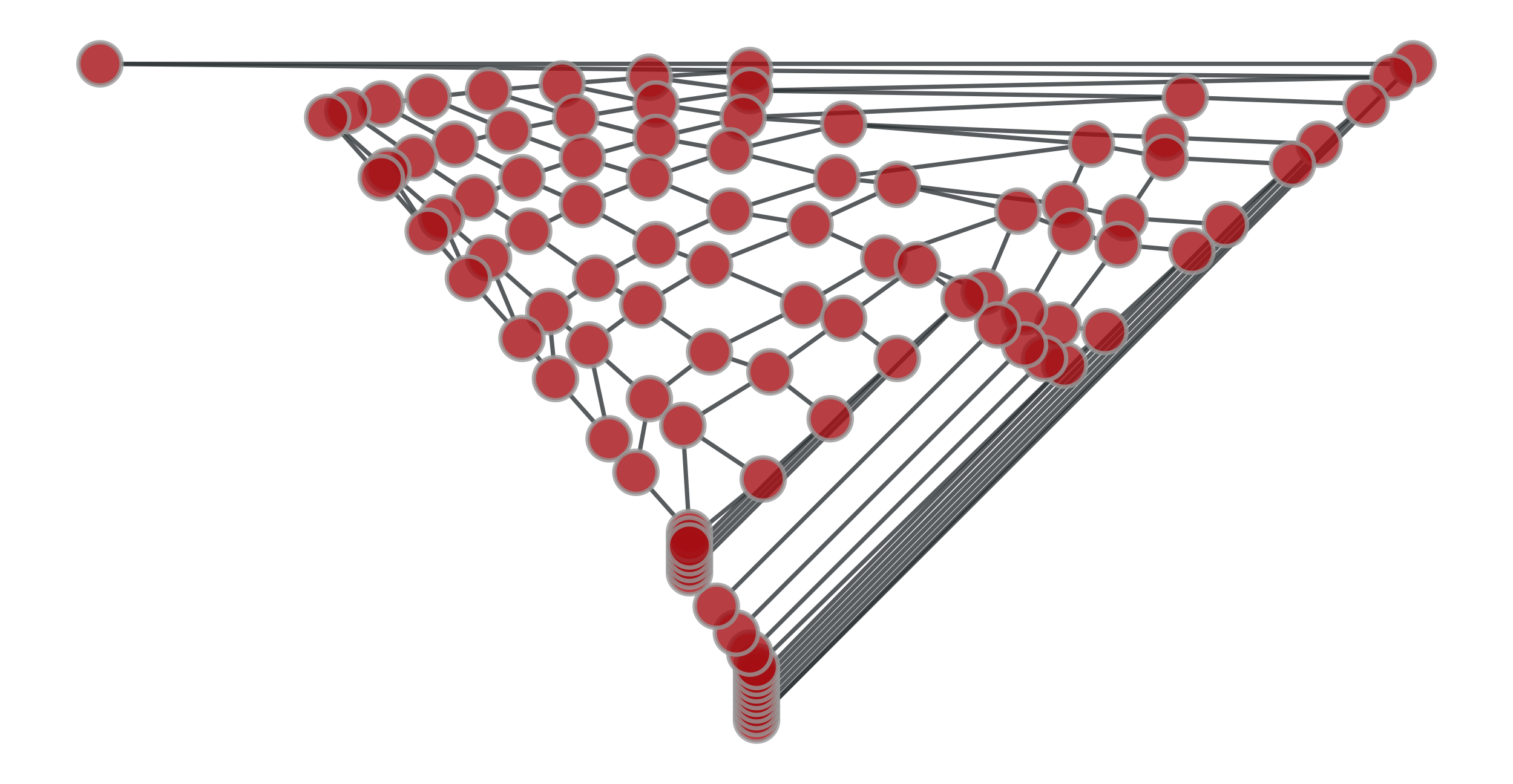Straight-line drawing of planar graph (a 2D square lattice).

graph_tool.draw.fruchterman_reingold_layout(g, weight=None, a=None, r=1.0, scale=None, circular=False, grid=True, t_range=None, n_iter=100, pos=None)[source]

Calculate the Fruchterman-Reingold spring-block layout of the graph.

Parameters
gGraph

Graph to be used.

weightEdgePropertyMap (optional, default: None)

An edge property map with the respective weights.

afloat (optional, default: $$V$$)

Attracting force between adjacent vertices.

rfloat (optional, default: 1.0)

Repulsive force between vertices.

scalefloat (optional, default: $$\sqrt{V}$$)

Total scale of the layout (either square side or radius).

circularbool (optional, default: False)

If True, the layout will have a circular shape. Otherwise the shape will be a square.

gridbool (optional, default: True)

If True, the repulsive forces will only act on vertices which are on the same site on a grid. Otherwise they will act on all vertex pairs.

t_rangetuple of floats (optional, default: (scale / 10, scale / 1000))

Temperature range used in annealing. The temperature limits the displacement at each iteration.

n_iterint (optional, default: 100)

Total number of iterations.

posVertexPropertyMap (optional, default: None)

Vector vertex property maps where the coordinates should be stored. If provided, this will also be used as the initial position of the vertices.

Returns
posVertexPropertyMap

A vector-valued vertex property map with the coordinates of the vertices.

Notes

This algorithm is defined in [fruchterman-reingold], and has complexity $$O(\text{n-iter}\times V^2)$$ if grid=False or $$O(\text{n-iter}\times (V + E))$$ otherwise.

References

fruchterman-reingold(1,2)

Fruchterman, Thomas M. J.; Reingold, Edward M. “Graph Drawing by Force-Directed Placement”. Software - Practice & Experience (Wiley) 21 (11): 1129-1164. (1991) DOI: 10.1002/spe.4380211102 [sci-hub, @tor]

Examples

>>> g = gt.price_network(300)
>>> pos = gt.fruchterman_reingold_layout(g, n_iter=1000)
>>> gt.graph_draw(g, pos=pos, output="graph-draw-fr.pdf")
<...>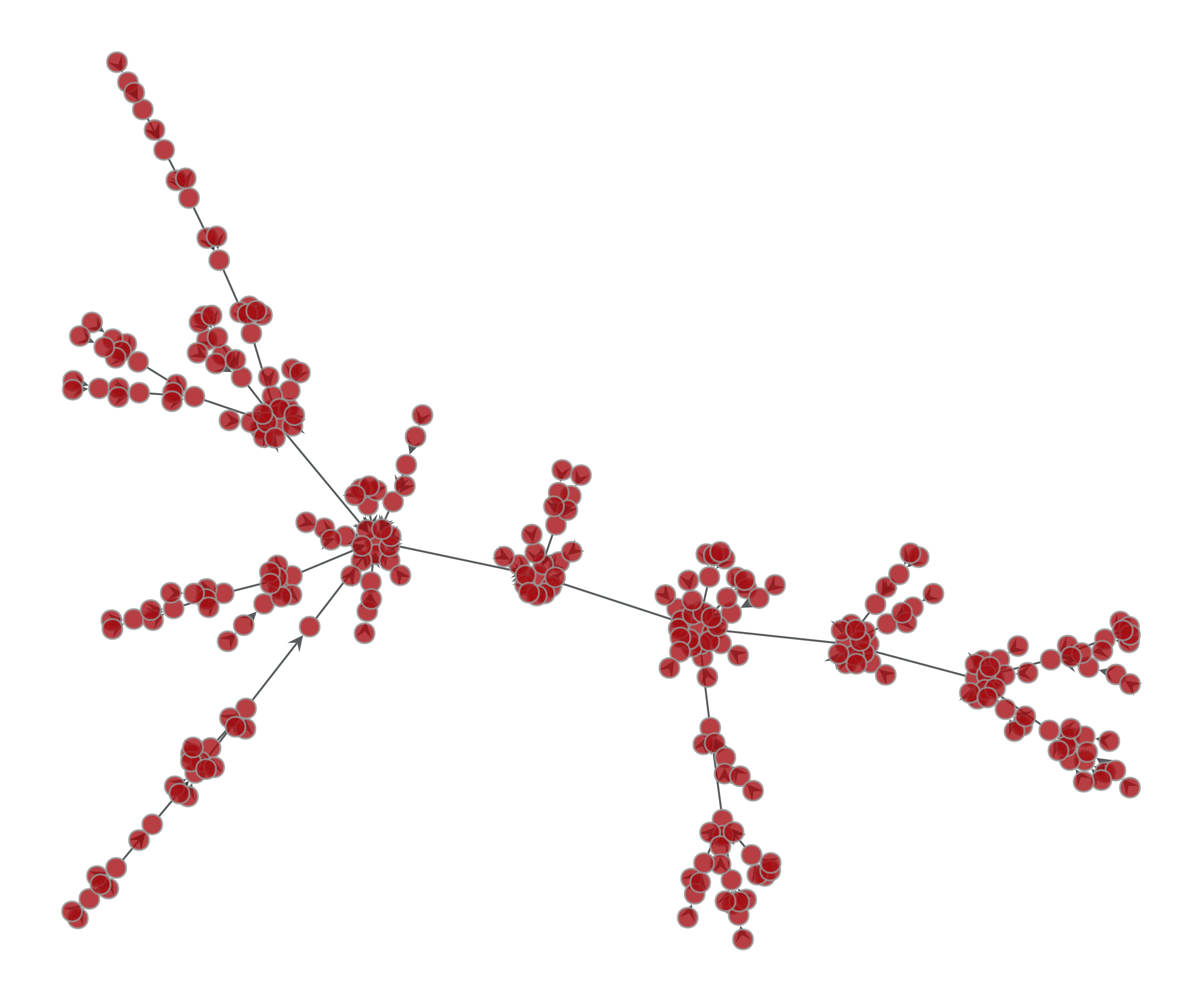Fruchterman-Reingold layout of a Price network.

graph_tool.draw.arf_layout(g, weight=None, d=0.5, a=10, dt=0.001, epsilon=1e-06, max_iter=1000, pos=None, dim=2)[source]

Calculate the ARF spring-block layout of the graph.

Parameters
gGraph

Graph to be used.

weightEdgePropertyMap (optional, default: None)

An edge property map with the respective weights.

dfloat (optional, default: 0.5)

Opposing force between vertices.

afloat (optional, default: 10)

Attracting force between adjacent vertices.

dtfloat (optional, default: 0.001)

Iteration step size.

epsilonfloat (optional, default: 1e-6)

Convergence criterion.

max_iterint (optional, default: 1000)

Maximum number of iterations. If this value is 0, it runs until convergence.

posVertexPropertyMap (optional, default: None)

Vector vertex property maps where the coordinates should be stored.

dimint (optional, default: 2)

Number of coordinates per vertex.

Returns
posVertexPropertyMap

A vector-valued vertex property map with the coordinates of the vertices.

Notes

This algorithm is defined in [geipel-self-organization-2007], and has complexity $$O(V^2)$$.

References

geipel-self-organization-2007(1,2)

Markus M. Geipel, “Self-Organization applied to Dynamic Network Layout”, International Journal of Modern Physics C vol. 18, no. 10 (2007), pp. 1537-1549, DOI: 10.1142/S0129183107011558 [sci-hub, @tor], arXiv: 0704.1748v5

Examples

>>> g = gt.price_network(300)
>>> pos = gt.arf_layout(g, max_iter=0)
>>> gt.graph_draw(g, pos=pos, output="graph-draw-arf.pdf")
<...>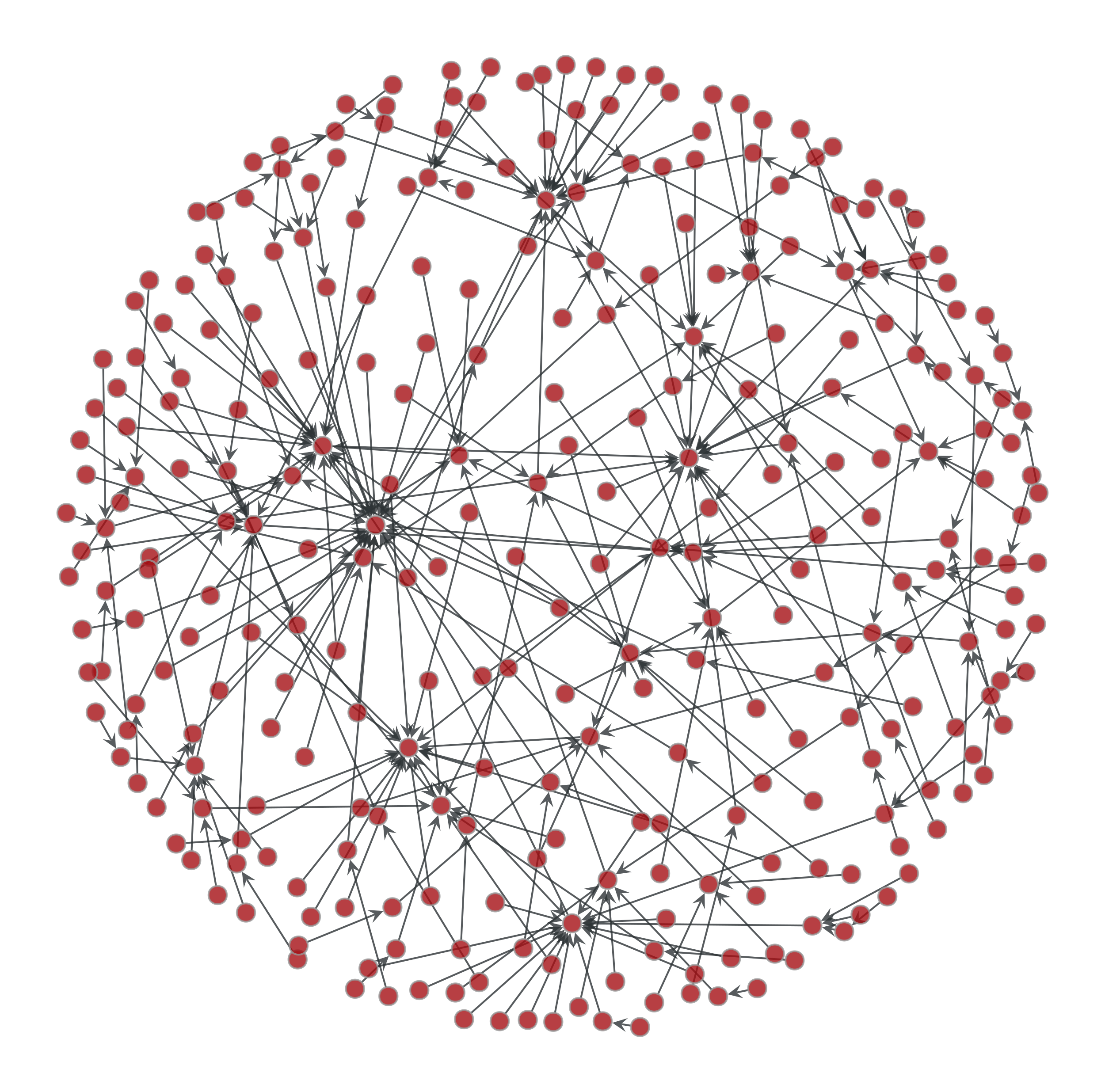ARF layout of a Price network.

graph_tool.draw.sfdp_layout(g, vweight=None, eweight=None, pin=None, groups=None, C=0.2, K=None, p=2.0, theta=0.6, max_level=15, gamma=1.0, mu=0.0, mu_p=1.0, init_step=None, cooling_step=0.95, adaptive_cooling=True, epsilon=0.01, max_iter=0, pos=None, multilevel=None, coarse_method='hybrid', mivs_thres=0.9, ec_thres=0.75, coarse_stack=None, weighted_coarse=False, verbose=False)[source]

Obtain the SFDP spring-block layout of the graph.

Parameters
gGraph

Graph to be used.

vweightVertexPropertyMap (optional, default: None)

A vertex property map with the respective weights.

eweightEdgePropertyMap (optional, default: None)

An edge property map with the respective weights.

pinVertexPropertyMap (optional, default: None)

A vertex property map with boolean values, which, if given, specify the vertices which will not have their positions modified.

groupsVertexPropertyMap (optional, default: None)

A vertex property map with group assignments. Vertices belonging to the same group will be put close together.

Cfloat (optional, default: 0.2)

Relative strength of repulsive forces.

Kfloat (optional, default: None)

Optimal edge length. If not provided, it will be taken to be the average edge distance in the initial layout.

pfloat (optional, default: 2)

Repulsive force exponent.

thetafloat (optional, default: 0.6)

Quadtree opening parameter, a.k.a. Barnes-Hut opening criterion.

max_levelint (optional, default: 15)

gammafloat (optional, default: 1.0)

Strength of the attractive force between connected components, or group assignments.

mufloat (optional, default: 0.0)

Strength of the attractive force between vertices of the same connected component, or group assignment.

mu_pfloat (optional, default: 1.0)

Scaling exponent of the attractive force between vertices of the same connected component, or group assignment.

init_stepfloat (optional, default: None)

Initial update step. If not provided, it will be chosen automatically.

cooling_stepfloat (optional, default: 0.95)

Cooling update step.

adaptive_coolingbool (optional, default: True)

Use an adaptive cooling scheme.

epsilonfloat (optional, default: 0.01)

Relative convergence criterion.

max_iterint (optional, default: 0)

Maximum number of iterations. If this value is 0, it runs until convergence.

posVertexPropertyMap (optional, default: None)

Initial vertex layout. If not provided, it will be randomly chosen.

multilevelbool (optional, default: None)

Use a multilevel layout algorithm. If None is given, it will be activated based on the size of the graph.

coarse_methodstr (optional, default: "hybrid")

Coarsening method used if multilevel == True. Allowed methods are "hybrid", "mivs" and "ec".

mivs_thresfloat (optional, default: 0.9)

If the relative size of the MIVS coarse graph is above this value, the coarsening stops.

ec_thresfloat (optional, default: 0.75)

If the relative size of the EC coarse graph is above this value, the coarsening stops.

weighted_coarsebool (optional, default: False)

Use weighted coarse graphs.

verbosebool (optional, default: False)

Provide verbose information.

Returns
posVertexPropertyMap

A vector-valued vertex property map with the coordinates of the vertices.

Notes

This algorithm is defined in [hu-multilevel-2005], and has complexity $$O(V\log V)$$.

References

hu-multilevel-2005(1,2)

Yifan Hu, “Efficient and High Quality Force-Directed Graph”, Mathematica Journal, vol. 10, Issue 1, pp. 37-71, (2005) http://www.mathematica-journal.com/issue/v10i1/graph_draw.html

Examples

>>> g = gt.price_network(3000)
>>> pos = gt.sfdp_layout(g)
>>> gt.graph_draw(g, pos=pos, output="graph-draw-sfdp.pdf")
<...>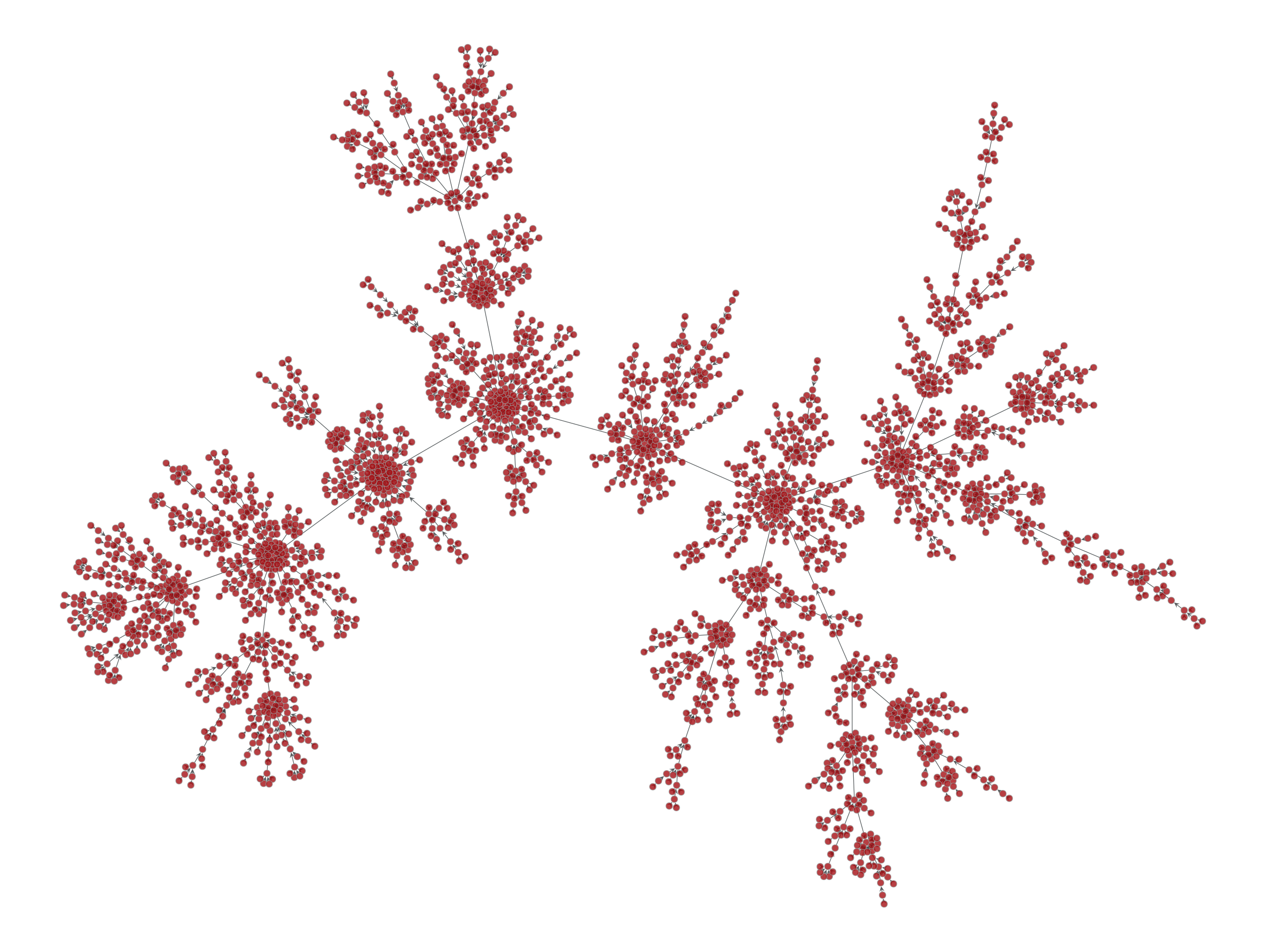SFDP layout of a Price network.

graph_tool.draw.radial_tree_layout(g, root, rel_order=None, rel_order_leaf=False, weighted=False, node_weight=None, r=1.0)[source]

Computes a radial layout of the graph according to the minimum spanning tree centered at the root vertex.

Parameters
gGraph

Graph to be used.

rootVertex or int

The root of the radial tree.

rel_orderVertexPropertyMap (optional, default: None)

Relative order of the nodes at each respective branch.

rel_order_leafbool (optional, default: False)

If True, the relative order of the leafs will propagate to the root. Otherwise they will propagate in the opposite direction.

weightedbool (optional, default: False)

If true, the angle between the child branches will be computed according to weight of the entire sub-branches.

node_weightVertexPropertyMap (optional, default: None)

If given, the relative spacing between leafs will correspond to the node weights.

rfloat (optional, default: 1.)

Layer spacing.

Returns
posVertexPropertyMap

A vector-valued vertex property map with the coordinates of the vertices.

Notes

This algorithm has complexity $$O(V + E)$$, or $$O(V\log V + E)$$ if rel_order is given.

Examples

>>> g = gt.price_network(1000)
>>> pos = gt.radial_tree_layout(g, g.vertex(0))
>>> gt.graph_draw(g, pos=pos, output="graph-draw-radial.pdf")
<...>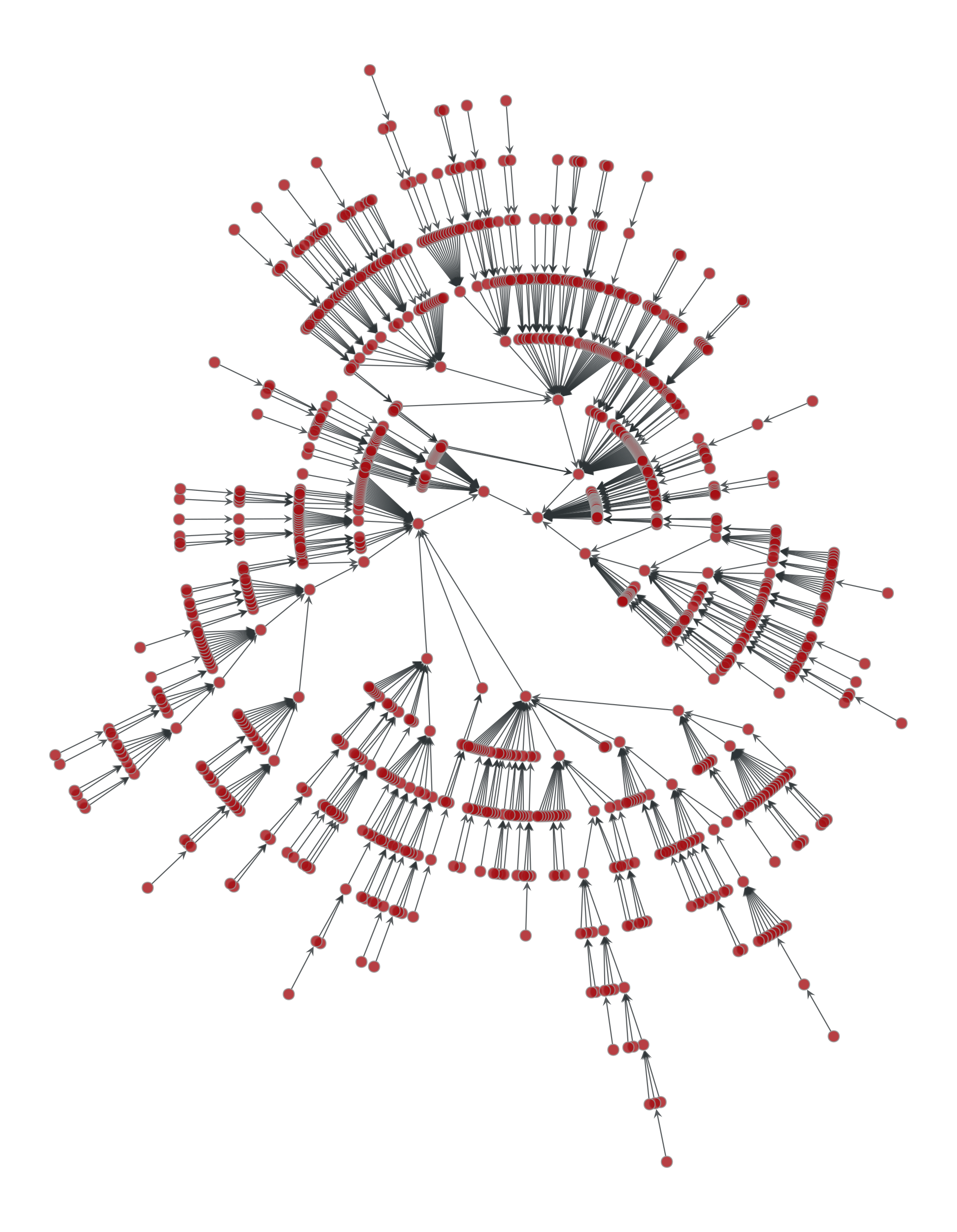Radial tree layout of a Price network.

graph_tool.draw.prop_to_size(prop, mi=0, ma=5, log=False, power=0.5)[source]

Convert property map values to be more useful as a vertex size, or edge width. The new values are taken to be

$y = mi + (ma - mi) \left(\frac{x_i - \min(x)} {\max(x) - \min(x)}\right)^\text{power}$

If log=True, the natural logarithm of the property values is used instead.

graph_tool.draw.graph_draw(g, pos=None, vprops=None, eprops=None, vorder=None, eorder=None, nodesfirst=False, output_size=(600, 600), fit_view=True, inline=False, mplfig=None, output=None, fmt='auto', **kwargs)[source]

Draw a graph to screen or to a file using cairo.

Parameters
gGraph

Graph to be drawn.

posVertexPropertyMap (optional, default: None)

Vector-valued vertex property map containing the x and y coordinates of the vertices. If not given, it will be computed using sfdp_layout().

vpropsdict (optional, default: None)

Dictionary with the vertex properties. Individual properties may also be given via the vertex_<prop-name> parameters, where <prop-name> is the name of the property.

epropsdict (optional, default: None)

Dictionary with the edge properties. Individual properties may also be given via the edge_<prop-name> parameters, where <prop-name> is the name of the property.

vorderVertexPropertyMap (optional, default: None)

If provided, defines the relative order in which the vertices are drawn.

eorderEdgePropertyMap (optional, default: None)

If provided, defines the relative order in which the edges are drawn.

nodesfirstbool (optional, default: False)

If True, the vertices are drawn first, otherwise the edges are.

output_sizetuple of scalars (optional, default: (600,600))

Size of the drawing canvas. The units will depend on the output format (pixels for the screen, points for PDF, etc).

fit_viewbool, float or tuple (optional, default: True)

If True, the layout will be scaled to fit the entire clip region. If a float value is given, it will be interpreted as True, and in addition the viewport will be scaled out by that factor. If a tuple value is given, it should have four values (x, y, w, h) that specify the view in user coordinates.

inlinebool (optional, default: False)

If True and an IPython notebook is being used, an inline version of the drawing will be returned.

mplfigmatplotlib container object (optional, default: None)

The mplfig object needs to have an artists attribute. This can for example be a matplotlib.figure.Figure or matplotlib.axes.Axes. Only the cairo backend is supported; use switch_backend('cairo').

outputstring or file object (optional, default: None)

Output file name (or object). If not given, the graph will be displayed via interactive_window().

fmtstring (default: "auto")

Output file format. Possible values are "auto", "ps", "pdf", "svg", and "png". If the value is "auto", the format is guessed from the output parameter.

bg_colorstr or sequence (optional, default: None)

Background color. The default is transparent.

vertex_*VertexPropertyMap or arbitrary types (optional, default: None)

Parameters following the pattern vertex_<prop-name> specify the vertex property with name <prop-name>, as an alternative to the vprops parameter.

edge_*EdgePropertyMap or arbitrary types (optional, default: None)

Parameters following the pattern edge_<prop-name> specify the edge property with name <prop-name>, as an alternative to the eprops parameter.

**kwargs

Any extra parameters are passed to interactive_window(), GraphWindow, GraphWidget and cairo_draw().

Returns
posVertexPropertyMap

Vector vertex property map with the x and y coordinates of the vertices.

selectedVertexPropertyMap (optional, only if output is None)

Boolean-valued vertex property map marking the vertices which were selected interactively.

Notes

List of vertex properties

Name

Description

Accepted types

Default Value

shape

The vertex shape. Can be one of the following strings: “circle”, “triangle”, “square”, “pentagon”, “hexagon”, “heptagon”, “octagon” “double_circle”, “double_triangle”, “double_square”, “double_pentagon”, “double_hexagon”, “double_heptagon”, “double_octagon”, “pie”, “none”. Optionally, this might take a numeric value corresponding to position in the list above.

str or int

"circle"

color

Color used to stroke the lines of the vertex.

str or list of floats

[0., 0., 0., 1]

fill_color

Color used to fill the interior of the vertex.

str or list of floats

[0.640625, 0, 0, 0.9]

size

The size of the vertex, in the default units of the output format (normally either pixels or points).

float or int

5

aspect

The aspect ratio of the vertex.

float or int

1.0

rotation

Angle (in radians) to rotate the vertex.

float

0.

anchor

Specifies how the edges anchor to the vertices. If 0, the anchor is at the center of the vertex, otherwise it is at the border.

int

1

pen_width

Width of the lines used to draw the vertex, in the default units of the output format (normally either pixels or points).

float or int

0.8

halo

Whether to draw a circular halo around the vertex.

bool

False

halo_color

Color used to draw the halo.

str or list of floats

[0., 0., 1., 0.5]

halo_size

Relative size of the halo.

float

1.5

text

Text to draw together with the vertex.

str

""

text_color

Color used to draw the text. If the value is "auto", it will be computed based on fill_color to maximize contrast.

str or list of floats

"auto"

text_position

Position of the text relative to the vertex. If the passed value is positive, it will correspond to an angle in radians, which will determine where the text will be placed outside the vertex. If the value is negative, the text will be placed inside the vertex. If the value is -1, the vertex size will be automatically increased to accommodate the text. The special value "centered" positions the texts rotated radially around the center of mass.

float or int

or "centered"

-1

text_offset

Text position offset.

list of float

[0.0, 0.0]

text_rotation

Angle of rotation (in radians) for the text. The center of rotation is the position of the vertex.

float

0.0

font_family

Font family used to draw the text.

str

"serif"

font_slant

Font slant used to draw the text.

cairo.FONT_SLANT_*

cairo.FONT_SLANT_NORMAL

font_weight

Font weight used to draw the text.

cairo.FONT_WEIGHT_*

cairo.FONT_WEIGHT_NORMAL

font_size

Font size used to draw the text.

float or int

12

surface

The cairo surface used to draw the vertex. If the value passed is a string, it is interpreted as an image file name to be loaded.

cairo.Surface or str

None

pie_fractions

Fractions of the pie sections for the vertices if shape=="pie".

list of int or float

[0.75, 0.25]

pie_colors

Colors used in the pie sections if shape=="pie".

list of strings or float.

('b','g','r','c','m','y','k')

List of edge properties

Name

Description

Accepted types

Default Value

color

Color used to stroke the edge lines.

str or list of floats

[0.179, 0.203,0.210, 0.8]

pen_width

Width of the line used to draw the edge, in the default units of the output format (normally either pixels or points).

float or int

1.0

start_marker, mid_marker, end_marker

Edge markers. Can be one of “none”, “arrow”, “circle”, “square”, “diamond”, or “bar”. Optionally, this might take a numeric value corresponding to position in the list above.

str or int

none

mid_marker_pos

Relative position of the middle marker.

float

0.5

marker_size

Size of edge markers, in units appropriate to the output format (normally either pixels or points).

float or int

4

control_points

Control points of a Bézier spline used to draw the edge. Each spline segment requires 6 values corresponding to the (x,y) coordinates of the two intermediary control points and the final point.

sequence of floats

[]

Stop points of a linear gradient used to stroke the edge. Each group of 5 elements is interpreted as [o, r, g, b, a] where o is the offset in the range [0, 1] and the remaining values specify the colors.

sequence of floats

[]

dash_style

Dash pattern is specified by an array of positive values. Each value provides the length of alternate “on” and “off” portions of the stroke. The last value specifies an offset into the pattern at which the stroke begins.

sequence of floats

[]

text

Text to draw next to the edges.

str

""

text_color

Color used to draw the text.

str or list of floats

[0., 0., 0., 1.]

text_distance

Distance from the edge and its text.

float or int

4

text_parallel

If True the text will be drawn parallel to the edges.

bool

True

font_family

Font family used to draw the text.

str

"serif"

font_slant

Font slant used to draw the text.

cairo.FONT_SLANT_*

cairo.FONT_SLANT_NORMAL

font_weight

Font weight used to draw the text.

cairo.FONT_WEIGHT_*

cairo.FONT_WEIGHT_NORMAL

font_size

Font size used to draw the text.

float or int

12

Examples

>>> g = gt.price_network(1500)
>>> deg = g.degree_property_map("in")
>>> deg.a = 4 * (sqrt(deg.a) * 0.5 + 0.4)
>>> ebet = gt.betweenness(g)
>>> ebet.a /= ebet.a.max() / 10.
>>> eorder = ebet.copy()
>>> eorder.a *= -1
>>> pos = gt.sfdp_layout(g)
>>> control = g.new_edge_property("vector<double>")
>>> for e in g.edges():
...     d = sqrt(sum((pos[e.source()].a - pos[e.target()].a) ** 2)) / 5
...     control[e] = [0.3, d, 0.7, d]
>>> gt.graph_draw(g, pos=pos, vertex_size=deg, vertex_fill_color=deg, vorder=deg,
...               edge_color=ebet, eorder=eorder, edge_pen_width=ebet,
...               edge_control_points=control, # some curvy edges
...               output="graph-draw.pdf")
<...>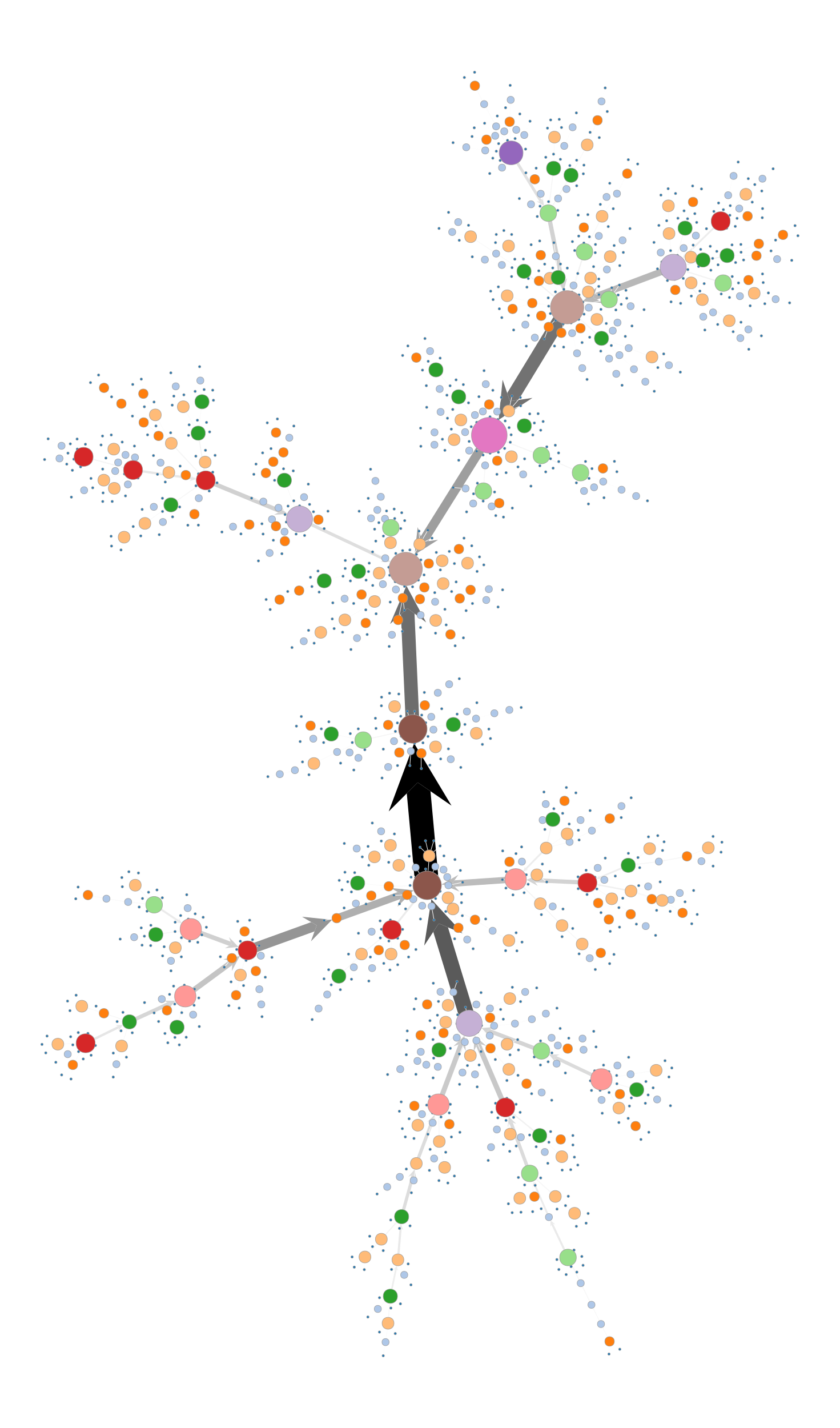SFDP force-directed layout of a Price network with 1500 nodes. The vertex size and color indicate the degree, and the edge color and width the edge betweenness centrality.

graph_tool.draw.graphviz_draw(g, pos=None, size=(15, 15), pin=False, layout=None, maxiter=None, ratio='fill', overlap=True, sep=None, splines=False, vsize=0.105, penwidth=1.0, elen=None, gprops={}, vprops={}, eprops={}, vcolor='#a40000', ecolor='#2e3436', vcmap=None, vnorm=True, ecmap=None, enorm=True, vorder=None, eorder=None, output='', output_format='auto', fork=False, return_string=False)[source]

Draw a graph using graphviz.

Parameters
gGraph

Graph to be drawn.

posVertexPropertyMap or tuple of VertexPropertyMap (optional, default: None)

Vertex property maps containing the x and y coordinates of the vertices.

sizetuple of scalars (optional, default: (15,15))

Size (in centimeters) of the canvas.

pinbool or VertexPropertyMap (default: False)

If True, the vertices are not moved from their initial position. If a VertexPropertyMap is passed, it is used to pin nodes individually.

layoutstring (default: "neato" if g.num_vertices() <= 1000 else "sfdp")

Layout engine to be used. Possible values are "neato", "fdp", "dot", "circo", "twopi" and "arf".

maxiterint (default: None)

If specified, limits the maximum number of iterations.

ratiostring or float (default: "fill")

Sets the aspect ratio (drawing height/drawing width) for the drawing. Note that this is adjusted before the size attribute constraints are enforced.

If ratio is numeric, it is taken as the desired aspect ratio. Then, if the actual aspect ratio is less than the desired ratio, the drawing height is scaled up to achieve the desired ratio; if the actual ratio is greater than that desired ratio, the drawing width is scaled up.

If ratio == "fill" and the size attribute is set, node positions are scaled, separately in both x and y, so that the final drawing exactly fills the specified size.

If ratio == "compress" and the size attribute is set, dot attempts to compress the initial layout to fit in the given size. This achieves a tighter packing of nodes but reduces the balance and symmetry. This feature only works in dot.

If ratio == "expand", the size attribute is set, and both the width and the height of the graph are less than the value in size, node positions are scaled uniformly until at least one dimension fits size exactly. Note that this is distinct from using size as the desired size, as here the drawing is expanded before edges are generated and all node and text sizes remain unchanged.

If ratio == "auto", the page attribute is set and the graph cannot be drawn on a single page, then size is set to an “ideal” value. In particular, the size in a given dimension will be the smallest integral multiple of the page size in that dimension which is at least half the current size. The two dimensions are then scaled independently to the new size. This feature only works in dot.

overlapbool or string (default: "prism")

Determines if and how node overlaps should be removed. Nodes are first enlarged using the sep attribute. If True, overlaps are retained. If the value is "scale", overlaps are removed by uniformly scaling in x and y. If the value is False, node overlaps are removed by a Voronoi-based technique. If the value is "scalexy", x and y are separately scaled to remove overlaps.

If sfdp is available, one can set overlap to "prism" to use a proximity graph-based algorithm for overlap removal. This is the preferred technique, though "scale" and False can work well with small graphs. This technique starts with a small scaling up, controlled by the overlap_scaling attribute, which can remove a significant portion of the overlap. The prism option also accepts an optional non-negative integer suffix. This can be used to control the number of attempts made at overlap removal. By default, overlap == "prism" is equivalent to overlap == "prism1000". Setting overlap == "prism0" causes only the scaling phase to be run.

If the value is "compress", the layout will be scaled down as much as possible without introducing any overlaps, obviously assuming there are none to begin with.

sepfloat (default: None)

Specifies margin to leave around nodes when removing node overlap. This guarantees a minimal non-zero distance between nodes.

splinesbool (default: False)

If True, the edges are drawn as splines and routed around the vertices.

vsizefloat, VertexPropertyMap, or tuple (default: 0.105)

Default vertex size (width and height). If a tuple is specified, the first value should be a property map, and the second is a scale factor.

penwidthfloat, EdgePropertyMap or tuple (default: 1.0)

Specifies the width of the pen, in points, used to draw lines and curves, including the boundaries of edges and clusters. It has no effect on text. If a tuple is specified, the first value should be a property map, and the second is a scale factor.

elenfloat or EdgePropertyMap (default: None)

Preferred edge length, in inches.

gpropsdict (default: {})

Additional graph properties, as a dictionary. The keys are the property names, and the values must be convertible to string.

vpropsdict (default: {})

Additional vertex properties, as a dictionary. The keys are the property names, and the values must be convertible to string, or vertex property maps, with values convertible to strings.

epropsdict (default: {})

Additional edge properties, as a dictionary. The keys are the property names, and the values must be convertible to string, or edge property maps, with values convertible to strings.

vcolorstring or VertexPropertyMap (default: "#a40000")

Drawing color for vertices. If the valued supplied is a property map, the values must be scalar types, whose color values are obtained from the vcmap argument.

ecolorstring or EdgePropertyMap (default: "#2e3436")

Drawing color for edges. If the valued supplied is a property map, the values must be scalar types, whose color values are obtained from the ecmap argument.

vcmapmatplotlib.colors.Colormap (default: matplotlib.cm.jet)

Vertex color map.

vnormbool (default: True)

Normalize vertex color values to the [0,1] range.

ecmapmatplotlib.colors.Colormap (default: matplotlib.cm.jet)

Edge color map.

enormbool (default: True)

Normalize edge color values to the [0,1] range.

vorderPropertyMap (default: None)

Scalar vertex property map which specifies the order with which vertices are drawn.

eorderVertexPropertyMap (default: None)

Scalar edge property map which specifies the order with which edges are drawn.

outputstring (default: "")

Output file name.

output_formatstring (default: "auto")

Output file format. Possible values are "auto", "xlib", "ps", "svg", "svgz", "fig", "mif", "hpgl", "pcl", "png", "gif", "dia", "imap", "cmapx". If the value is "auto", the format is guessed from the output parameter, or xlib if it is empty. If the value is None, no output is produced.

forkbool (default: False)

If True, the program is forked before drawing. This is used as a work-around for a bug in graphviz, where the exit() function is called, which would cause the calling program to end. This is always assumed True, if output_format == 'xlib'.

return_stringbool (default: False)

If True, a string containing the rendered graph as binary data is returned (defaults to png format).

Returns
posVertexPropertyMap

Vector vertex property map with the x and y coordinates of the vertices.

gvgv.digraph or gv.graph (optional, only if returngv == True)

Internally used graphviz graph.

Notes

This function is a wrapper for the [graphviz] routines. Extensive additional documentation for the graph, vertex and edge properties is available at: http://www.graphviz.org/doc/info/attrs.html.

References

graphviz(1,2)

http://www.graphviz.org

Examples

>>> g = gt.price_network(1500)
>>> deg = g.degree_property_map("in")
>>> deg.a = 2 * (sqrt(deg.a) * 0.5 + 0.4)
>>> ebet = gt.betweenness(g)
>>> gt.graphviz_draw(g, vcolor=deg, vorder=deg, elen=10,
...                  ecolor=ebet, eorder=ebet, output="graphviz-draw.pdf")
<...>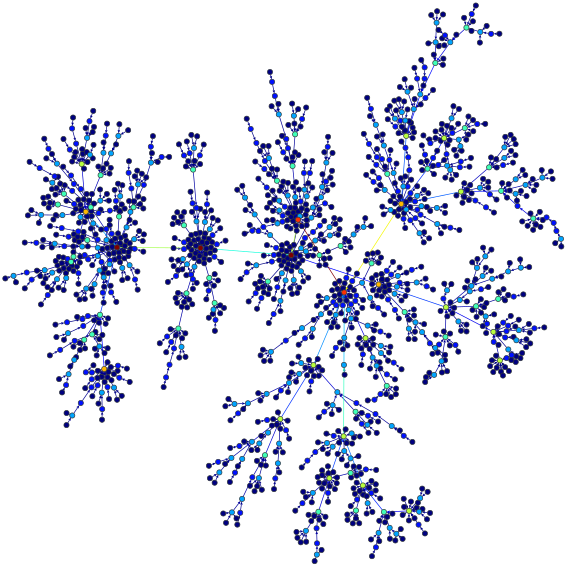Kamada-Kawai force-directed layout of a Price network with 1500 nodes. The vertex size and color indicate the degree, and the edge color corresponds to the edge betweenness centrality

graph_tool.draw.cairo_draw(g, pos, cr, vprops=None, eprops=None, vorder=None, eorder=None, nodesfirst=False, vcmap=<matplotlib.colors.LinearSegmentedColormap object>, ecmap=<matplotlib.colors.LinearSegmentedColormap object>, loop_angle=nan, parallel_distance=None, fit_view=False, res=0, max_render_time=-1, **kwargs)[source]

Draw a graph to a cairo context.

Parameters
gGraph

Graph to be drawn.

posVertexPropertyMap

Vector-valued vertex property map containing the x and y coordinates of the vertices.

crContext

A Context instance.

vpropsdict (optional, default: None)

Dictionary with the vertex properties. Individual properties may also be given via the vertex_<prop-name> parameters, where <prop-name> is the name of the property.

epropsdict (optional, default: None)

Dictionary with the edge properties. Individual properties may also be given via the edge_<prop-name> parameters, where <prop-name> is the name of the property.

vorderVertexPropertyMap (optional, default: None)

If provided, defines the relative order in which the vertices are drawn.

eorderEdgePropertyMap (optional, default: None)

If provided, defines the relative order in which the edges are drawn.

nodesfirstbool (optional, default: False)

If True, the vertices are drawn first, otherwise the edges are.

vcmapmatplotlib.colors.Colormap or tuple (optional, default: default_cm)

Vertex color map. Optionally, this may be a (matplotlib.colors.Colormap, alpha) tuple.

ecmapmatplotlib.colors.Colormap or tuple (optional, default: default_cm)

Edge color map. Optionally, this may be a (matplotlib.colors.Colormap, alpha) tuple.

loop_anglefloat or EdgePropertyMap (optional, default: nan)

Angle used to draw self-loops. If nan is given, they will be placed radially from the center of the layout.

parallel_distancefloat (optional, default: None)

Distance used between parallel edges. If not provided, it will be determined automatically.

fit_viewbool or float or tuple (optional, default: True)

If True, the layout will be scaled to fit the entire clip region. If a float value is given, it will be interpreted as True, and in addition the viewport will be scaled out by that factor. If a tuple value is given, it should have four values (x, y, w, h) that specify the view in user coordinates.

bg_colorstr or sequence (optional, default: None)

Background color. The default is transparent.

resfloat (optional, default: 0.):

If shape sizes fall below this value, simplified drawing is used.

max_render_timeint (optional, default: -1):

If nonnegative, this function will return an iterator that will perform part of the drawing at each step, so that each iteration takes at most max_render_time milliseconds.

vertex_*VertexPropertyMap or arbitrary types (optional, default: None)

Parameters following the pattern vertex_<prop-name> specify the vertex property with name <prop-name>, as an alternative to the vprops parameter.

edge_*EdgePropertyMap or arbitrary types (optional, default: None)

Parameters following the pattern edge_<prop-name> specify the edge property with name <prop-name>, as an alternative to the eprops parameter.

Returns
iterator :

If max_render_time is nonnegative, this will be an iterator that will perform part of the drawing at each step, so that each iteration takes at most max_render_time milliseconds.

graph_tool.draw.get_hierarchy_control_points(g, t, tpos, beta=0.8, cts=None, is_tree=True, max_depth=None)[source]

Return the Bézier spline control points for the edges in g, given the hierarchical structure encoded in graph t.

Parameters
gGraph

Graph to be drawn.

tGraph

Directed graph containing the hierarchy of g. It must be a directed tree with a single root. The direction of the edges point from the root to the leaves, and the vertices in t with index in the range $$[0, N-1]$$, with $$N$$ being the number of vertices in g, must correspond to the respective vertex in g.

tposVertexPropertyMap

Vector-valued vertex property map containing the x and y coordinates of the vertices in graph t.

betafloat (optional, default: 0.8 or EdgePropertyMap)

Edge bundling strength. For beta == 0 the edges are straight lines, and for beta == 1 they strictly follow the hierarchy. This can be optionally an edge property map, which specified a different bundling strength for each edge.

ctsEdgePropertyMap (optional, default: None)

Edge property map of type vector<double> where the control points will be stored.

is_treebool (optional, default: True)

If True, t must be a directed tree, otherwise it can be any connected graph.

max_depthint (optional, default: None)

If supplied, only the first max_depth bottom levels of the hierarchy will be used.

Returns
ctsEdgePropertyMap

Vector-valued edge property map containing the Bézier spline control points for the edges in g.

Notes

This is an implementation of the edge-bundling algorithm described in [holten-hierarchical-2006].

References

holten-hierarchical-2006(1,2)

Holten, D. “Hierarchical Edge Bundles: Visualization of Adjacency Relations in Hierarchical Data.”, IEEE Transactions on Visualization and Computer Graphics 12, no. 5, 741–748 (2006). DOI: 10.1109/TVCG.2006.147 [sci-hub, @tor]

Examples

>>> g = gt.collection.data["netscience"]
>>> g = gt.GraphView(g, vfilt=gt.label_largest_component(g))
>>> g.purge_vertices()
>>> state = gt.minimize_nested_blockmodel_dl(g, deg_corr=True)
>>> t = gt.get_hierarchy_tree(state)
>>> tpos = pos = gt.radial_tree_layout(t, t.vertex(t.num_vertices() - 1), weighted=True)
>>> cts = gt.get_hierarchy_control_points(g, t, tpos)
>>> pos = g.own_property(tpos)
>>> b = state.levels.b
>>> shape = b.copy()
>>> shape.a %= 14
>>> gt.graph_draw(g, pos=pos, vertex_fill_color=b, vertex_shape=shape, edge_control_points=cts,
...               edge_color=[0, 0, 0, 0.3], vertex_anchor=0, output="netscience_nested_mdl.pdf")
<...>Block partition of a co-authorship network, which minimizes the description length of the network according to the nested (degree-corrected) stochastic blockmodel.

graph_tool.draw.interactive_window(g, pos=None, vprops=None, eprops=None, vorder=None, eorder=None, nodesfirst=False, geometry=(500, 400), update_layout=True, sync=True, main=True, **kwargs)[source]

Display an interactive GTK+ window containing the given graph.

Parameters
gGraph

Graph to be drawn.

posVertexPropertyMap (optional, default: None)

Vector-valued vertex property map containing the x and y coordinates of the vertices. If not given, it will be computed using sfdp_layout().

vpropsdict (optional, default: None)

Dictionary with the vertex properties. Individual properties may also be given via the vertex_<prop-name> parameters, where <prop-name> is the name of the property.

epropsdict (optional, default: None)

Dictionary with the vertex properties. Individual properties may also be given via the edge_<prop-name> parameters, where <prop-name> is the name of the property.

vorderVertexPropertyMap (optional, default: None)

If provided, defines the relative order in which the vertices are drawn.

eorderEdgePropertyMap (optional, default: None)

If provided, defines the relative order in which the edges are drawn.

nodesfirstbool (optional, default: False)

If True, the vertices are drawn first, otherwise the edges are.

geometrytuple (optional, default: (500, 400))

Window geometry.

update_layoutbool (optional, default: True)

If True, the layout will be updated dynamically.

syncbool (optional, default: True)

If False, run asynchronously. (Requires IPython)

mainbool (optional, default: True)

If False, the GTK+ main loop will not be called.

**kwargs

Any extra parameters are passed to GraphWindow, GraphWidget and cairo_draw().

Returns
posVertexPropertyMap

Vector vertex property map with the x and y coordinates of the vertices.

selectedVertexPropertyMap (optional, only if output is None)

Boolean-valued vertex property map marking the vertices which were selected interactively.

Notes

See documentation of GraphWidget for key bindings information.

class graph_tool.draw.GraphWidget(g, pos, vprops=None, eprops=None, vorder=None, eorder=None, nodesfirst=False, update_layout=False, layout_K=1.0, multilevel=False, display_props=None, display_props_size=11, fit_view=True, bg_color=None, max_render_time=300, layout_callback=None, key_press_callback=None, highlight_color=None, **kwargs)[source]

Bases: gi.repository.Gtk.DrawingArea

Interactive GTK+ widget displaying a given graph.

Parameters
gGraph

Graph to be drawn.

posVertexPropertyMap

Vector-valued vertex property map containing the x and y coordinates of the vertices.

vpropsdict (optional, default: None)

Dictionary with the vertex properties. Individual properties may also be given via the vertex_<prop-name> parameters, where <prop-name> is the name of the property.

epropsdict (optional, default: None)

Dictionary with the edge properties. Individual properties may also be given via the edge_<prop-name> parameters, where <prop-name> is the name of the property.

vorderVertexPropertyMap (optional, default: None)

If provided, defines the relative order in which the vertices are drawn.

eorderEdgePropertyMap (optional, default: None)

If provided, defines the relative order in which the edges are drawn.

nodesfirstbool (optional, default: False)

If True, the vertices are drawn first, otherwise the edges are.

update_layoutbool (optional, default: False)

If True, the layout will be updated dynamically.

layout_Kfloat (optional, default: 1.0)

Parameter K passed to sfdp_layout().

multilevelbool (optional, default: False)

Parameter multilevel passed to sfdp_layout().

display_propslist of VertexPropertyMap instances (optional, default: None)

List of properties to be displayed when the mouse passes over a vertex.

display_props_sizefloat (optional, default: 11.)

Font size used to display the vertex properties.

fit_viewbool, float or tuple (optional, default: True)

If True, the layout will be scaled to fit the entire clip region. If a float value is given, it will be interpreted as True, and in addition the viewport will be scaled out by that factor. If a tuple value is given, it should have four values (x, y, w, h) that specify the view in user coordinates.

bg_colorstr or sequence (optional, default: None)

Background color. The default is white.

max_render_timeint (optional, default: 300)

Maximum amount of time (in milliseconds) spent rendering portions of the graph.

layout_callbackfunction (optional, default: Node)

User-supplied callback to be called whenever the positions of the layout have changed. It needs to have the following signature:

def callback(g, picked, pos, vprops, eprops):
...


where g is the graph being drawn, picked is either a single vertex or a boolean vertex property map representing the vertices currently selected, and vprops and eprops are dictionaries with the vertex and edge properties currently being used by the layout.

key_press_callbackfunction (optional, default: Node)

User-supplied callback to be called whenever a key-press event has happened. It needs to have the following signature:

def callback(g, keyval, picked, pos, vprops, eprops):
...


where g is the graph being drawn, keyval is the key id, picked is either a single vertex or a boolean vertex property map representing the vertices currently selected, and vprops and eprops are dictionaries with the vertex and edge properties currently being used by the layout.

vertex_*VertexPropertyMap or arbitrary types (optional, default: None)

Parameters following the pattern vertex_<prop-name> specify the vertex property with name <prop-name>, as an alternative to the vprops parameter.

edge_*EdgePropertyMap or arbitrary types (optional, default: None)

Parameters following the pattern edge_<prop-name> specify the edge property with name <prop-name>, as an alternative to the eprops parameter.

**kwargs

Any extra parameters are passed to cairo_draw().

Notes

The graph drawing can be panned by dragging with the middle mouse button pressed. The graph may be zoomed by scrolling with the mouse wheel, or equivalent (if the “shift” key is held, the vertex/edge sizes are scaled accordingly). The layout may be rotated by dragging while holding the “control” key. Pressing the “r” key centers and zooms the layout around the graph. By pressing the “a” key, the current translation, scaling and rotation transformations are applied to the vertex positions themselves, and the transformation matrix is reset (if this is never done, the given position properties are never modified).

Individual vertices may be selected by pressing the left mouse button. The currently selected vertex follows the mouse pointer. To stop the selection, the right mouse button must be pressed. Alternatively, a group of vertices may be selected by holding the “shift” button while the pointer is dragged while pressing the left button. The selected vertices may be moved by dragging the pointer with the left button pressed. They may be rotated by holding the “control” key and scrolling with the mouse. If the key “z” is pressed, the layout is zoomed to fit the selected vertices only.

If the key “s” is pressed, the dynamic spring-block layout is activated. Vertices which are currently selected are not updated.

cleanup()[source]

Cleanup callbacks.

reset_layout()[source]

Reset the layout algorithm.

layout_callback()[source]

Perform one step of the layout algorithm.

regenerate_surface(reset=False, complete=False)[source]

Redraw the graph surface.

draw(da, cr)[source]

Redraw the widget.

pos_to_device(pos, dist=False, surface=False, cr=None)[source]

Convert a position from the graph space to the widget space.

pos_from_device(pos, dist=False, surface=False, cr=None)[source]

Convert a position from the widget space to the device space.

apply_transform()[source]

Apply current transform matrix to vertex coordinates.

fit_to_window(ink=False, g=None)[source]

Fit graph to window.

init_picked()[source]

Init picked vertices.

button_press_event(widget, event)[source]

Handle button press.

button_release_event(widget, event)[source]

Handle button release.

motion_notify_event(widget, event)[source]

Handle pointer motion.

scroll_event(widget, event)[source]

Handle scrolling.

key_press_event(widget, event)[source]

Handle key press.

key_release_event(widget, event)[source]

Handle release event.

zoom_begin(gesture, seq)[source]
zoom_end(gesture, seq)[source]
scale_changed(gesture, scale)[source]
rotate_begin(gesture, seq)[source]
rotate_end(gesture, seq)[source]
angle_changed(gesture, angle, angle_delta)[source]
drag_gesture_begin(gesture, seq)[source]
drag_gesture_end(gesture, seq)[source]
drag_gesture_update(gesture, dx, dy)[source]
class graph_tool.draw.GraphWindow(g, pos, geometry, vprops=None, eprops=None, vorder=None, eorder=None, nodesfirst=False, update_layout=False, **kwargs)[source]

Bases: gi.overrides.Gtk.Window

Interactive GTK+ window containing a GraphWidget.

Parameters
gGraph

Graph to be drawn.

posVertexPropertyMap

Vector-valued vertex property map containing the x and y coordinates of the vertices.

geometrytuple

Widget geometry.

vpropsdict (optional, default: None)

Dictionary with the vertex properties. Individual properties may also be given via the vertex_<prop-name> parameters, where <prop-name> is the name of the property.

epropsdict (optional, default: None)

Dictionary with the edge properties. Individual properties may also be given via the edge_<prop-name> parameters, where <prop-name> is the name of the property.

vorderVertexPropertyMap (optional, default: None)

If provided, defines the relative order in which the vertices are drawn.

eorderEdgePropertyMap (optional, default: None)

If provided, defines the relative order in which the edges are drawn.

nodesfirstbool (optional, default: False)

If True, the vertices are drawn first, otherwise the edges are.

update_layoutbool (optional, default: True)

If True, the layout will be updated dynamically.

**kwargs

Any extra parameters are passed to GraphWidget and cairo_draw().

graph_tool.draw.draw_hierarchy(state, pos=None, layout='radial', beta=0.8, node_weight=None, vprops=None, eprops=None, hvprops=None, heprops=None, subsample_edges=None, rel_order='degree', deg_size=True, vsize_scale=1, hsize_scale=1, hshortcuts=0, hide=0, bip_aspect=1.0, empty_branches=False, **kwargs)[source]

Draw a nested block model state in a circular hierarchy layout with edge bundling.

Parameters
stateNestedBlockState

Nested block state to be drawn.

posVertexPropertyMap (optional, default: None)

If supplied, this specifies a vertex property map with the positions of the vertices in the layout.

layoutstr or VertexPropertyMap (optional, default: "radial")

If layout == "radial" radial_tree_layout() will be used. If layout == "sfdp", the hierarchy tree will be positioned using sfdp_layout(). If layout == "bipartite" a bipartite layout will be used. If instead a VertexPropertyMap is provided, it must correspond to the position of the hierarchy tree.

betafloat (optional, default: .8)

Edge bundling strength.

vpropsdict (optional, default: None)

Dictionary with the vertex properties. Individual properties may also be given via the vertex_<prop-name> parameters, where <prop-name> is the name of the property. See graph_draw() for details.

epropsdict (optional, default: None)

Dictionary with the edge properties. Individual properties may also be given via the edge_<prop-name> parameters, where <prop-name> is the name of the property. See graph_draw() for details.

hvpropsdict (optional, default: None)

Dictionary with the vertex properties for the hierarchy tree. Individual properties may also be given via the hvertex_<prop-name> parameters, where <prop-name> is the name of the property. See graph_draw() for details.

hepropsdict (optional, default: None)

Dictionary with the edge properties for the hierarchy tree. Individual properties may also be given via the hedge_<prop-name> parameters, where <prop-name> is the name of the property. See graph_draw() for details.

subsample_edgesint or list of Edge instances (optional, default: None)

If provided, only this number of random edges will be drawn. If the value is a list, it should include the edges that are to be drawn.

rel_orderstr or None or VertexPropertyMap (optional, default: "degree")

If degree, the vertices will be ordered according to degree inside each group, and the relative ordering of the hierarchy branches. If instead a VertexPropertyMap is provided, its value will be used for the relative ordering.

deg_sizebool (optional, default: True)

If True, the (total) node degrees will be used for the default vertex sizes..

vsize_scalefloat (optional, default: 1.)

Multiplicative factor for the default vertex sizes.

hsize_scalefloat (optional, default: 1.)

Multiplicative factor for the default sizes of the hierarchy nodes.

hshortcutsint (optional, default: 0)

Include shortcuts to the number of upper layers in the hierarchy determined by this parameter.

hideint (optional, default: 0)

Hide upper levels of the hierarchy.

bip_aspectfloat (optional, default: 1.)

If layout == "bipartite", this will define the aspect ratio of layout.

empty_branchesbool (optional, default: False)

If empty_branches == False, dangling branches at the upper layers will be pruned.

vertex_*VertexPropertyMap or arbitrary types (optional, default: None)

Parameters following the pattern vertex_<prop-name> specify the vertex property with name <prop-name>, as an alternative to the vprops parameter. See graph_draw() for details.

edge_*EdgePropertyMap or arbitrary types (optional, default: None)

Parameters following the pattern edge_<prop-name> specify the edge property with name <prop-name>, as an alternative to the eprops parameter. See graph_draw() for details.

hvertex_*VertexPropertyMap or arbitrary types (optional, default: None)

Parameters following the pattern hvertex_<prop-name> specify the vertex property with name <prop-name>, as an alternative to the hvprops parameter. See graph_draw() for details.

hedge_*EdgePropertyMap or arbitrary types (optional, default: None)

Parameters following the pattern hedge_<prop-name> specify the edge property with name <prop-name>, as an alternative to the heprops parameter. See graph_draw() for details.

**kwargs :

All remaining keyword arguments will be passed to the graph_draw() function.

Returns
posVertexPropertyMap

This is a vertex property map with the positions of the vertices in the layout.

tGraph

This is a the hierarchy tree used in the layout.

tposVertexPropertyMap

This is a vertex property map with the positions of the hierarchy tree in the layout.

References

holten-hierarchical-2006

Holten, D. “Hierarchical Edge Bundles: Visualization of Adjacency Relations in Hierarchical Data.”, IEEE Transactions on Visualization and Computer Graphics 12, no. 5, 741–748 (2006). DOI: 10.1109/TVCG.2006.147 [sci-hub, @tor]

Examples

>>> g = gt.collection.data["celegansneural"]
>>> state = gt.minimize_nested_blockmodel_dl(g, deg_corr=True)
>>> gt.draw_hierarchy(state, output="celegansneural_nested_mdl.pdf")
(...)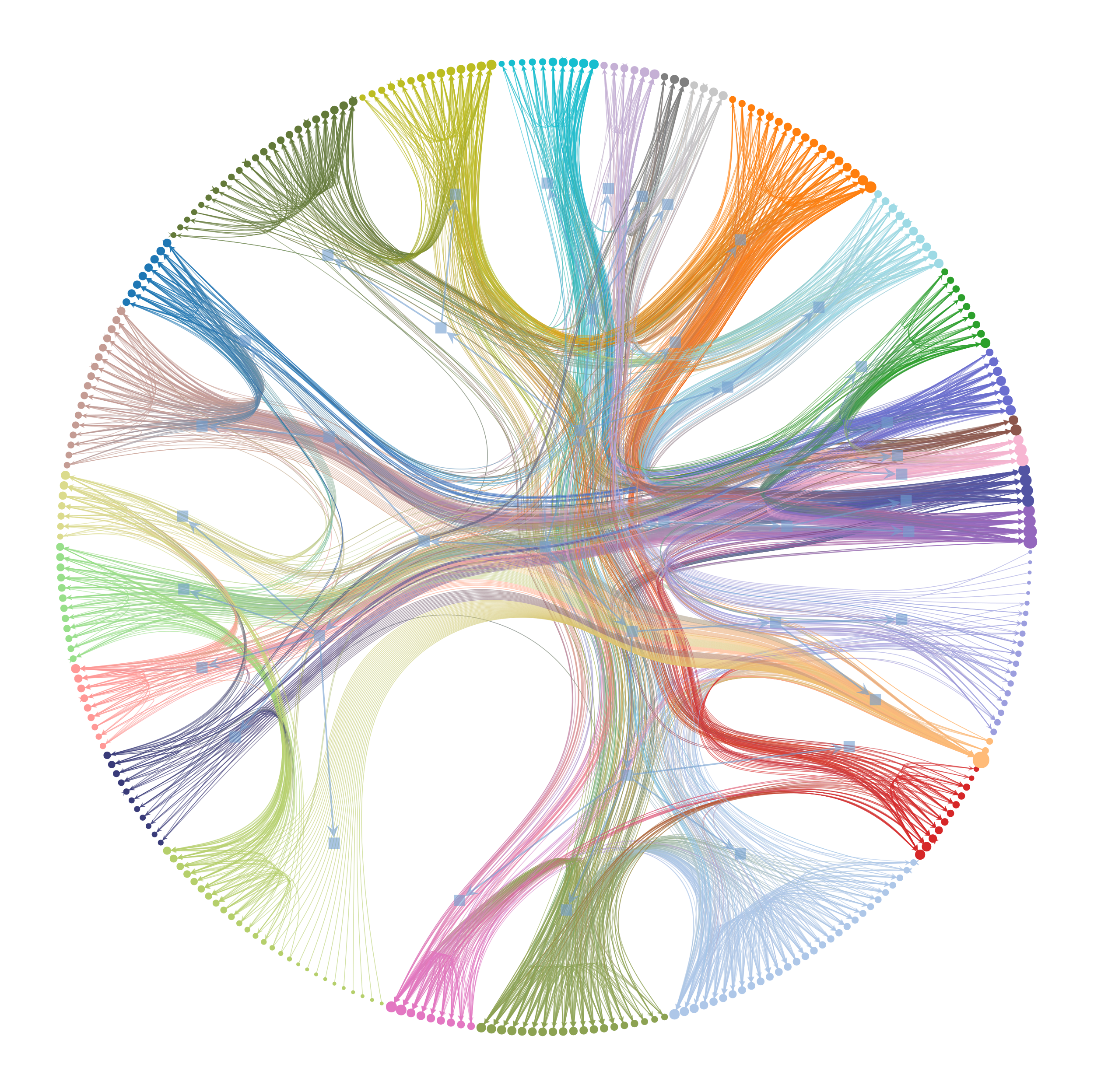Hierarchical block partition of the C. elegans neural network, which minimizes the description length of the network according to the nested (degree-corrected) stochastic blockmodel.